Name:    Chapter 2 Online Practice

Multiple Choice
Identify the letter of the choice that best completes the statement or answers the question.

1.

Which of the following postulates of Dalton's atomic theory are now known to be incorrect?

 1. Matter is made up of atoms. 2. All atoms of a given element are identical. 3. Atoms are indivisible and indestructible.
 a. 1 only b. 2 only c. 3 only d. 2 and 3 e. 1, 2, and 3

2.

The Millikan oil drop experiment determined
 a. the mass of a drop of oil. b. the charge on an electron. c. the charge to mass ratio of a proton. d. the mass of a neutron. e. the number of electrons in a drop of oil.

3.

Which of the following particles will not be deflected by charged plates?
 a. g particles b. b particles c. a particles d. protons e. a and b particles

4.

J. J. Thomson determined the charge to mass ratio of electrons by
 a. creating a charge on the amber by rubbing it with a cloth. b. deflecting cathode rays with magnetic and electric fields. c. exposing photographic plates to radioactive uranium ores. d. striking a beryllium target with particles emitted from radioactive polonium. e. bombarding gold foil with alpha particles.

5.

From the results of his gold foil experiment, Ernest Rutherford concluded that
 a. electrons have a charge of -1.602 ´ 10-19 C. b. atoms contain equal numbers of protons and electrons. c. uranium ores emit a form of radiation that affect photographic plates. d. alpha particles are helium nuclei. e. atoms are composed of a small, dense nucleus surrounded by a cloud of electrons.

6.

Beta (b) particles are identical to ____.
 a. neutrons b. electrons c. helium nuclei d. light e. protons

7.

Rank the subatomic particles from lowest to highest mass.
 a. electrons = protons < neutrons b. electrons < neutrons < protons c. electrons < protons < neutrons d. neutrons < electrons < protons e. electrons < protons = neutrons

8.

An atomic mass unit (u) is defined as
 a. the mass of one hydrogen-1 atom. b. 1/8 the mass of one oxygen-16 atom. c. 1/12 the mass of one carbon-12 atom. d. 1.99 ´ 10-23 g. e. the sum of the masses of one proton, one neutron, and one electron.

9.

How many protons, neutrons, and electrons are in an oxygen-18 atom?
 a. 6 protons, 8 neutrons, 4 electron b. 6 protons, 10 neutrons, 8 electrons c. 8 protons, 8 neutrons, 8 electrons d. 8 protons, 10 neutrons, 8 electrons e. 8 protons, 10 neutrons, 18 electrons

10.

What is the atomic symbol for an element with 28 protons and 31 neutrons?
 a.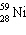b.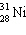c.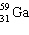d.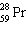e.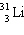11.

What is the identity of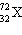?
 a. Hf b. Zr c. Rf d. Ge e. Cu

12.

Which of the following atoms contains the largest number of protons?
 a. 158Gd b. 157Tb c. 127I d. 138Ba e. 144Nd

13.

Which two of the atoms below have the same number of neutrons?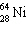,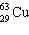,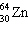,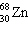,?
 a.andb.andc.andd.ande.and14.

All of the following statements are true EXCEPT
 a. for any neutral element, the number of protons and electrons are equal. b. electrons and protons have equal mass, but opposite charges. c. the mass number is the sum of the number of protons and neutrons. d. the atomic number equals the number of protons. e. isotopes of an element have identical atomic numbers.

15.

Which two of the following atoms are isotopes?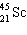,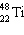,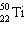,a.and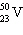b.andc.andd.ande.and16.

Bromine has two naturally occurring isotopes. The average mass of bromine is 79.904 u. If 50.54% of bromine is found as bromine-79 (78.9183 u), what is the mass of the other isotope?
 a. 79.82 u b. 79.97 u c. 80.91 u d. 81.93 u e. 82.91 u

17.

You have 0.330 mole of each of the following elements: Be, B, Br, Ba, and Bi. Which sample has the largest mass?
 a. Be b. B c. Br d. Ba e. Bi

18.

You have 4.15 g of each of the following elements: Ca, Cu, Ce, Cs, Cf. Which sample contains the largest number of atoms?
 a. Ca b. Cu c. Ce d. Cs e. Cf

19.

Calculate the number of moles in 0.41 g titanium.
 a. 9.1 ´ 10-4 mol b. 8.6 ´ 10-3 mol c. 0.051 mol d. 2.0 ´ 101 mol e. 1.2 ´ 102 mol

20.

What is the mass of 5.1 mol P?
 a. 6.3 ´ 10-3 g b. 1.6 ´ 10-1 g c. 6.1 g d. 1.6 ´ 102 g e. 2.0 ´ 102 g

21.

The molar mass of silver is 107.9 g/mol. What is the mass of a single silver atom?
 a. 1.539 ´ 10-26 g b. 1.792 ´ 10-22 g c. 1.079 ´ 10-20 g d. 1.901 ´ 10-18 g e. 5.581 ´ 1021 g

22.

The density of lithium is 0.546 g/cm3. What volume is occupied by 1.96 ´ 1023 atoms of lithium?
 a. 0.0859 cm3 b. 0.596 cm3 c. 4.14 cm3 d. 5.63 cm3 e. 39.0 cm3

23.

Which three elements are likely to have similar chemical and physical properties?
 a. sodium, lithium, and potassium b. sodium, magnesium, and aluminum c. nitrogen, oxygen, and neon d. nickel, copper, and zinc e. uranium, plutonium, and americium

24.

Structural isomers are compounds that have
 a. the same molar masses, but different elemental composition. b. have identical structures, but contain different isotopes of the same elements. c. two or more double bonds. d. the same elemental composition, but different molar masses. e. the same elemental composition, but the atoms are linked in different ways.

25.

What is the general formula for an alkene?
 a. CnH2n+2 b. CnH2n c. CnHn-2 d. Cn+2Hn e. CnH2n-2

26.

Which of the following molecules might be an alkyne?
 a. C2H6 b. C4H8 c. C5H8 d. C6H12 e. C6H14

27.

What is the molecular formula for heptane?
 a. C6H12 b. C6H14 c. C7H14 d. C7H16 e. C8H14

28.

What is the name of the following compound?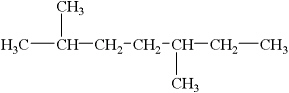a. 2,5-dimethylheptane b. 2,5-diheptane c. 2-ethyl-5-methylhexane d. 3,6-dimethylheptane e. 1,1,4-trimethylhexane

29.

What is the name of the following compound?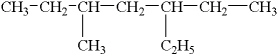a. 3,5-diethylmethylheptane b. 3-ethyl-5-methylheptane c. decane d. 2,4-diethylhexane e. 3-ethyl-5-methyldecane

30.

What is the name of the following compound?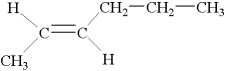a. 1-methyl-2-pentene b. cis-1-methyl-2-pentene c. trans-2-hexene d. cis-4-hexene e. trans-1-methyl-2-propylethylene

31.

What is the name of the following compound?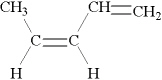32.

What is the molecular formula of this hydrocarbon?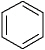a. C6 b. C6H6 c. C6H8 d. C6H12 e. C6H14

33.

Chalcopyrite is a copper containing ore. This ore contains 1 iron atom and 2 sulfur atoms for each copper atom. What is the formula of chalcopyrite?
 a. CuFeS b. CuFe(2S) c. CuFeS2 d. 2SCuFe e. CuFeS2

34.

Acetylsalicylic acid, commonly known as aspirin, has 9 carbon atoms, 8 hydrogen atoms, and 4 oxygen atoms per molecule. What is the molecular formula of aspirin?
 a. C9He8O4 b. C9H8O4 c. C9H8Ox4 d. Ca9H8O2 e. C9(HO4)2

35.

A nitride ion has ____ electrons.
 a. 2 b. 4 c. 7 d. 8 e. 10

36.

An aluminum ion has ____ electrons.
 a. 9 b. 10 c. 12 d. 13 e. 16

37.

Identify the ions present in KHCO3.
 a. KHCO3 is not ionic. b. KH+, and CO3- c. K+, H+, C4+, and O2- d. KH2+ and CO32- e. K+ and HCO3-

38.

Which atom is most likely to form a 1- ion?
 a. I b. S c. Ag d. P e. Na

39.

Which atom is most likely to form a 3+ ion?
 a. Li b. Sr c. Kr d. Fe e. P

40.

For a nonmetal in Group 5A of the periodic table, the most common monatomic ion will have a charge of ____.
 a. 3- b. 2- c. 1- d. 1+ e. 2+

41.

Which of the following formulas is not correct?
 a. Al2(SO4)3 b. NaClO3 c. Ba2O3 d. Mg(NO3)2 e. KH2PO4

42.

What is the correct formula for an ionic compound that contains aluminum ions and carbonate ions?
 a. AlCO3 b. Al(CO3)2 c. Al(CO3)3 d. Al2(CO3)3 e. Al3(CO3)2

43.

What is the correct formula for an ionic compound that contains magnesium ions and fluoride ions?
 a. Mg2F b. MgF c. Mg2F2 d. MgF2 e. Mg2F3

44.

What is the charge on the lead ion in PbS2?
 a. 4- b. 2- c. 0 d. 2+ e. 4+

45.

What is the correct formula for sodium acetate?
 a. Na2CH3O b. NaCH3CO2 c. NaCH3O d. NaCH2O2 e. NaCHO

46.

What is the correct formula for potassium dihydrogen phosphate?
 a. K2H2PO4 b. KH2PO4 c. KH2PO3 d. K2H2P e. KH2P

47.

What is the correct formula for copper(II) chloride?
 a. Cu2Cl b. CuCl c. CuCl2 d. CuCl3 e. Cu2Cl2

48.

All of the following are named correctly EXCEPT
 a. LiClO4; lithium perchlorate. b. CaHPO4; calcium hydrogen phosphide. c. NaCN; sodium cyanide. d. Mg(OH)2; magnesium hydroxide. e. CaSO3; calcium sulfite.

49.

All of the following are named correctly EXCEPT
 a. NiCl2·6H2O; nickel(II) chloride hexahydrate. b. MnO2; manganese(IV) oxide. c. TiBr4; titanium(IV) bromide. d. AuI; gold(I) iodide. e. FeSO4; iron(I) sulfate.

50.

What is the correct name for NH4ClO4?
 a. ammonia hydrogen chlorate b. ammonia hydrogen perchlorate c. ammonium perchlorate d. ammonium hypochlorite e. ammonium hydrochloric acid

51.

What is the correct name for Cr(NO3)2?
 a. chromium(II) nitrate b. chromium(II) dinitrate c. chromium dinitrate d. dinitrochromate e. chromium(II) nitride

52.

What is the correct name for SO2?
 a. sulfur oxide b. sulfur dioxide c. sulfuric dioxide d. sulfuric acid e. sulfurous oxide

53.

What is the correct name for PF5?
 a. phosphorus pentafluoride b. phosphorus pentafluorine c. phosphorus(V) fluoride d. phosphorus fluorate e. phosphorus fluorine

54.

What is the molar mass of cobalt(II) iodide hexahydrate?
 a. 212.8 g/mol b. 293.9 g/mol c. 312.7 g/mol d. 420.8 g/mol e. 465.1 g/mol

55.

How many moles are in 4.5 g of AgNO3?
 a. 1.3 ´ 10-3 mol b. 2.6 ´ 10-2 mol c. 0.61 mol d. 38 mol e. 7.6 ´ 102 mol

56.

How many oxygen atoms are in 1.50 mol of SO3?
 a. 7.71 ´ 1021 atoms b. 1.12 ´ 1022 atoms c. 3.01 ´ 1022 atoms d. 9.03 ´ 1023 atoms e. 2.71 ´ 1024 atoms

57.

What is the mass percent of each element in dichloromethane, CH2Cl2?
 a. 10.06% C, 60.24% H, 29.70% Cl b. 20.00% C, 20.00% H, 60.00% Cl c. 24.10% C, 3.11% H, 72.79% Cl d. 33.87% C, 0.22% H, 65.91% Cl e. 14.14% C, 2.37% H, 83.48% Cl

58.

Nitrogen and oxygen form an extensive series of oxides with the general formula NxOy. What is the empirical formula for an oxide that contains 46.68% nitrogen?
 a. N2O b. NO c. NO2 d. N2O3 e. N2O5

59.

Phosphorus, P, is combined with chlorine, Cl2, to give a gaseous compound with the formula PClx. If you start with 2.097 g of P and isolate 9.298 g of PClx, what is the value of x?
 a. 2 b. 3 c. 4 d. 5 e. 6